# Movable cellular automaton

﻿
Movable cellular automaton
Method type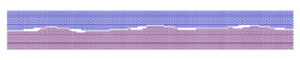Movable cellular automaton actively changed self neighbors by means switching neighbors state from linked to unlinked and vice versa (modeling of contact interaction) Discrete Computational cellular automaton, discrete element computational solid mechanics

The Movable cellular automaton (MCA) method is a method in computational solid mechanics based on the discrete concept. It provides advantages both of classical cellular automaton and discrete element methods. Important advantage of the МСА method is a possibility of direct simulation of materials fracture including damage generation, crack propagation, fragmentation and mass mixing. It is difficult to simulate these processes by means of continuum mechanics methods (For example: finite element method, finite difference method, etc.), so some new concepts like peridynamics is required. Discrete element method is very effective to simulate granular materials, but mutual forces among movable cellular automata provides simulating solids behavior. If size of automaton will be close to zero then MCA behavior becomes like classical continuum mechanics methods.

## Keystone of the movable cellular automaton method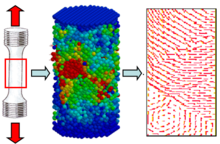Object (at left) is described as set of interacted automata (at center). At right is shown velocity field of automata.

In framework of the MCA approach an object under modeling is considered as a set of interacting elements/automata. The dynamics of the set of automata are defined by their mutual forces and rules for their relationships. This system exists and operates in time and space. Its evolution in time and space is governed by the equations of motion. The mutual forces and rules for inter-elements relationships are defined by the function of the automaton response. This function has to be specified for each automaton. Due to mobility of automata the following new parameters of cellular automata have to be included into consideration: Ri – radius-vector of automaton; Vi – velocity of automaton; ωi – rotation velocity of automaton; θi – rotation vector of automaton; mi – mass of automaton; Ji – moment of inertia of automaton.

## New concept: neighboursEach automaton has some neighbors

The new concept of the MCA method is based on the introducing of the state of the pair of automata (relation of interacting pairs of automata) in addition to the conventional one – the state of a separate automaton. Note that the introduction of this definition allows to go from the static net concept to the concept of neighbours. As a result of this, the automata have the ability to change their neighbors by switching the states (relationships) of the pairs.

## Definition of the parameter of pair state

The introducing of new type of states leads to new parameter to use it as criteria for switching relationships. It is defined as an automaton overlapping parameters hij. So the relationship of the cellular automata is characterised by the value of their overlapping.The initial structure is formed by setting up certain relationships among each pair of neighboring elements.

## Criterion of switching of the state of pair relationships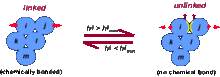At left pair of automata ij is linked. At right pair of automata ij is unlinked.

In contrast to the classical cellular automaton method in the MCA method not only a single automaton but also a relationship of pair of automata can be switched. According with the bistable automata concept there are two types of the pair states (relationships):

 linked – both automata belong to a solid unlinked – each automaton of the pair belongs to different bodies or parts of damaged body.

So the changing of the state of pair relationships is controlled by relative movements of the automata and the media formed by such pairs can be considered as bistable media.

## Equations of MCA motion

The evolution of MCA media is described by the following equations of motion for translation:${d^2 h^{ij} \over dt^2} = \left( {1 \over m^i} + {1 \over m^j} \right) p^{ij} + \sum_{k\neq j} C(ij,ik) \psi(\alpha_{ij,ik}) {1 \over m^i} p^{ik} + \sum_{l \neq i} C(ij,jl) \psi(\alpha_{ij,jl}) {1 \over m^j} p^{jl}$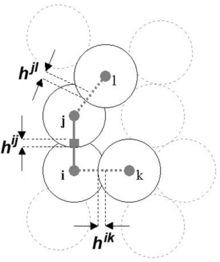Forces between automata ij coming from their neighbors.

Here mi is the mass of automaton i, pij is central force acting between automata i and j, C(ij,ik) is certain coefficient associated with transferring the h parameter from pair ij to pair ik, ψ(αij,ik) is angle between directions ij and ik.

Due to finite size of movable automata the rotation effects have to be taken into account. The equations of motion for rotation can be written as follows:${d^2 \theta^{ij} \over dt^2} = \left( {q^{ij} \over J^i} + {q^{ji} \over J^j} \right) \tau^{ij} + \sum_{k\neq j} S(ij,ik) {q^{ik} \over J^i} \tau^{ik} + \sum_{l\neq j} S(ij,jl) {q^{jl} \over J^j} \tau^{jl}$

Here Θij is the angle of relative rotation (it is a switching parameter like hij for translation), qij is the distance from center of automaton i to contact point of automaton j (moment arm), τij is the pair tangential interaction, S(ij,ik) is certain coefficient associated with transferring the Θ parameter from one pair to other (it is similar to C(ij,ik) from the equation for translation).

It should be noted that these equations are completely similar to the equations of motion for the many–particle approach.

## Definition of deformation in pair of automataRotation of body as whole not caused to deformation in pair of automata

Translation of the pair automata The dimensionless deformation parameter for translation of the i j automata pair can be presented as:$\varepsilon^{ij} = {h^{ij} \over r_{0}^{ij}} = { \left( q^{ij} + q^{ji} \right) - \left( d^{i} + d^{j} \right) \big / 2 \over \left( d^{i} + d^{j} \right) \big / 2 }$

In this case:$\left( \Delta{\varepsilon^{i(j)}} + \Delta{\varepsilon^{j(i)}} \right) { \left( d^{i} + d^{j} \right) \over 2} = V_{n}^{ij} \Delta{t}$

where Δt time step, Vnij – relative velocity.

Rotation of the pair automata can be calculated by analogy with the last translation relationships.

## Modeling of irreversible deformation in the MCA method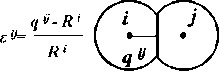Deformation is determine by value of distance from the center of automaton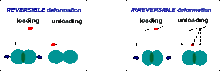There are two types of the response function of automata

The εij parameter is used as a measure of deformation of automaton i under its interaction with automaton j. Where qij – is a distance from the center of automaton i to its contact point with automaton j; Ri = di/2 (di – is the size of automaton i).

As an example the titanium specimen under cyclic loading (tension – compression) is considered. The loading diagram is shown in the next figure:(Red marks are the experimental data)

Due to mobility of each automaton the MCA method allows to take into account directly such actions as:

• mass mixing
• penetration effects
• chemical reactions
• intensive deformation
• phase transformations
• accumulation of damages
• fragmentation and fracture
• cracks generation and development

Using boundary conditions of different types (fixed, elastic, viscous-elastic, etc.) it is possible to imitate different properties of surrounding medium, containing the simulated system. It is possible to model different modes of mechanical loading (tension, compression, shear strain, etc.) by setting up additional conditions at the boundaries.

Wikimedia Foundation. 2010.

### Look at other dictionaries:

• Continuum mechanics — Continuum mechanics …   Wikipedia

• MCA — may refer to: Contents 1 Archaeology 2 Aviation 3 Chemistry 4 …   Wikipedia

• Solid mechanics — Continuum mechanics …   Wikipedia

• Computational mechanics — For the scientific journal, see Computational Mechanics. Computational mechanics is the discipline concerned with the use of computational methods to study phenomena governed by the principles of mechanics. Before the emergence of computational… …   Wikipedia

• MCA — MCA  многозначная аббревиатура, имеющая следующие расшифровки: Machine check architecture Maritime and Coastguard Agency  Агентство морской безопасности и береговой охраны Великобритании. Micro Channel Architecture (архитектура Micro… …   Википедия

• Physical Sciences — ▪ 2009 Introduction Scientists discovered a new family of superconducting materials and obtained unique images of individual hydrogen atoms and of a multiple exoplanet system. Europe completed the Large Hadron Collider, and China and India took… …   Universalium

• Traffic congestion — Traffic jam redirects here. For other uses, see Traffic jam (disambiguation). Traffic congestion on Marginal Pinheiros, near downtown São Paulo. According to Time magazine, São Paulo has the world s worst traffic jams.[1 …   Wikipedia

• Invention of the telephone — The modern telephone is the result of work done by many people, all worthy of recognition of their contributions to the field. Alexander Graham Bell was the first to patent the telephone, an apparatus for transmitting vocal or other sounds… …   Wikipedia